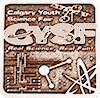BRONZE#### Eggs in salt water

I am gong to test how much salt you have to put in water for an egg to float in it.

### Hypothesis

I predict that if you add ¼ cup of salt (12 tsp) (130g), the egg will float.

### Research

Water has a density of one. To determine the density of the egg, we would have to first weigh the egg. Then, if we put the egg in a graduated cylinder filled with water and measured the amount of water displaced, we can find its exact volume. By dividing the mass by the volume we can find the density. The density of the average egg will be slightly greater than that of water, so it will sink. To make the egg float, we have to make the water more dense by adding salt. For 1 cup of water, adding 12 tsp/130.2g of salt should be about enough to make the egg float.

### Variables

Controlled:

For the controlled variables we have eggs and water. The do not change throughout the experiment, and stay at a constant level.

Manipulated:

Our manipulated variable is the salt concentration in the water.

Responding:

The responding variable is how well the egg floats. This will change depending on our manipulated variable.

### Procedure

1. Fill up a cup of water
2. Put an egg into the water
3. Put in a teaspoon of salt
4. Repeat step 3 until the egg floats

### Observations

 Amount of salt in teaspoons Mass of salt in grams Concentration g/ml Float 4 43.4 0.1 Lifted from bottom 5 54.25 0.125 Lifted from bottom 6 65.1 0.15 Lifted from bottom 7 75.95 0.175 Lifted from bottom 8 86.8 0.2 Lifted from bottom 9 97.65 0.225 Got close to top 10 108.5 0.25 Got close to top 11 119.35 0.275 It floated

### Analysis

Specific gravity of salt is 2.17 g/ml

1 cup is 237 ml

¼ cup is 59 ml

The mass of a ¼ cup of salt is 59 ml x 2.17 g/ml= 128g

The concentration is 128g/237ml= 0.54 g/ml

1 tsp= 5 ml

1 teaspoon of salt is 2.17 g/ml x 5 ml= 10.85g

The number of teaspoons is 128g/10.85g= 11.7g, which is about 12 teaspoons

### Conclusion

In conclusion, my hypothesis predicted that a ¼ cup of salt which is approximately 12 teaspoons, (128 g) was needed to make the egg float. The data shows that the egg only needed 11 teaspoons (119.35 g) to float. Therefore, my hypothesis was very close, less than 1 teaspoon difference.

### Application

A practical application for this experiment would be that you can see how salty the water is. For example, if you want to see how salty a lake is, a simple way would be to take about a cup of the water and put an egg in it and see if it floats. If it does, then the water is salty. If it doesnt, there is probably not much salt in the water.

### Sources Of Error

When I did the experiment I did not realize that the salt would displace the water, and I used a small cup so I had to change the cup to a bigger one in the middle of the experiment. Because of this, the results might have been a bit off.